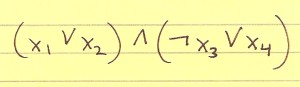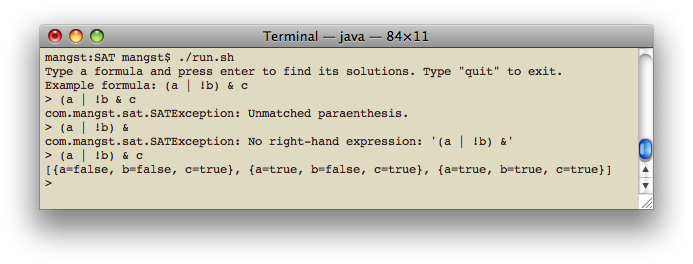< Back to Projects

# Boolean Satisfiability (SAT) Solver## Overview

A SAT formula contains a number of variables whose values can either be true or false. The operators AND, OR, and NOT are used in conjunction with the variables. A SAT solver finds out what the variables have to be in order for the entire formula to evaluate to true.

For example, the formula `(a | !b) & c` has three solutions:

1. `a=false, b=false, c=true`
2. `a=true, b=false, c=true`
3. `a=true, b=true, c=true`

SAT is an NP-Complete problem because as the number of inputs grow, the time it takes to solve the problem grows exponentially. For example, with 3 variables there are 2^3=8 possible solutions; with 4 variables there are 2^4=16 possible solutions; with 5 there are 2^5=32; and so on. Commercial SAT solvers have clever ways of pruning the search tree in order to more quickly solve the given problem (as opposed to using brute force and trying every single possibility). However, the problem is still technically NP-Complete.

## Requirements

Java 1.5 to run the program.
JUnit 4 to run the unit tests.